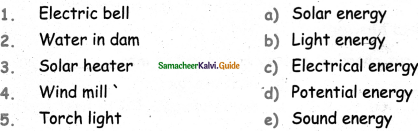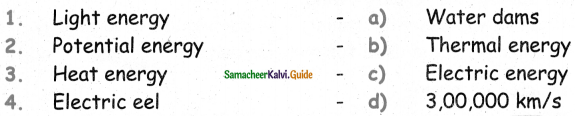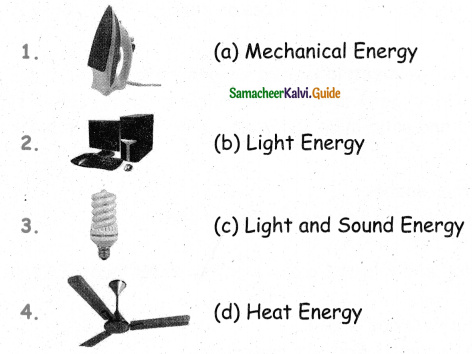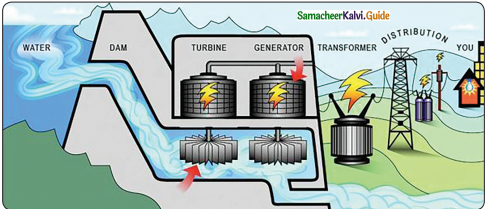Tamilnadu State Board New Syllabus Samacheer Kalvi 5th Science Guide Pdf Term 1 Chapter 3 Energy Text Book Back Questions and Answers, Notes.

## Tamilnadu Samacheer Kalvi 5th Science Solutions Term 1 Chapter 3 Energy

### Samacheer Kalvi 5th Science Guide Energy Text Book Back Questions and Answers

Evaluation

Question 1.
When diesel is burnt chemical energy is converted into _______.
a) wind energy
b) heat energy
c) solar energy
d) sound energy
b) heat energyQuestion 2.
Running water possesses _______.
a) potential energy
b) chemical energy
c) kinetic energy
d) sound energy
c) kinetic energy

Question 3.
Unit of energy is _______.
a) Kilogram
b) Newton
c) Kelvin
d) Joule
d) Joule

Question 4.
Which one of the following requires wind energy?
a) Bicycle
b) Photosynthesis
c) Parachute
d) Automobiles
c) Parachute

Question 5.
Cow dung possesses _______ .
a) kinetic energy
b) chemical energy
c) solar energy
d) heat energy
b) chemical energyII. Find out the energy conversion that takes place in the following:

Question 1.
Iron box: _______.
Chemical energy to Heat energy

Question 2.
Electric Iron box: _______.
Electric energy to Heat energy

Question 3.
Electric fan:
Electric energy to Mechanical energy

Question 4.
Speaker:
Electric energy to Sound energy

Question 5.
Generator: _______.
Mechanical energy to Electrical energyIII. Find out form of energy possessed by the following things:

Question 1.
A rock on the top of a hill.
Pontential Energy

Question 2.
A rolling ball.
Kinetic Energy

Question 3.
Charcoal.
Heat Energy

Question 4.
Water falls.
Kinetic Energy

Question 5.
Battery.
Chemical EnergyIV. Match the following:1. e
2. d
3. a
4. c
5. bV. Say True or False:

Question 1.
An apple falling from a tree is an example for kinetic energy.
True

Question 2.
Electrical energy is used to run electric trains.
True

Question 3.
Heat energy cannot be produced by friction.
False

Question 4.
Potential energy and heat energy are the two forms of mechanical energy.
False

Question 5.
The unit of energy is joule.
TrueQuestion 1.
What is energy?
Energy is defined as capacity to do work. S.I unit of work is Joule.

Question 2.
What are the different forms of energy?
There are different forms of energy like mechanical energy, heat energy, light energy, wind energy, solar energy, electrical energy, and chemical energy.

Question 3.
What are the uses of mechanical energy?

• In hydroelectric plants, kinetic energy of water is converted into electrical energy.
• Windmills convert kinetic energy of winds into electrical energy.
• Mechanical energy of the hammer is used to apply a force on a nail.
• Mechanical energy can bring a moving body to rest and make a body at rest to move.

Question 4.
State the Law of conservation of energy.
Law of conservation of energy states that energy Can neither be created nor be destroyed. One form of energy is converted into another form of energy.

Question 5.
Give the uses of Light energy.

• We are able to see objects with the help of light energy.
• Plants use light energy to synthesis their food.
• With the help of light energy, our skin is able to synthesis Vitamin D.
• Electricity can be produced with the help of light energy.Question 1.
Explain the types of Mechanical energy.
Energy possessed by an object due its position is called mechanical energy.
Mechanical energy can be classified into two;

• Kinetic energy
• Potential energy

a) Kinetic energy: Energy possessed by a moving objectis known as kinetic energy. It is also known as energy of motion.
Eg: Moving car, cricket ball bowled by a player, bullet coming out of a gun.

b) Potential energy: Energy possessed by an object which is at rest is known as potential energy. It is also known as stores energy of position.
Eg: Object lifted above, stone in the stretched rubber, water in the dam.

Question 2.
Explain Conservation of energy.
Energy cannot be created cannot be destroyed also. It is changed from one form to another form or transferred from one object to another object. Examples for conservation of energy in our daily life are :

1. Water dam :

• Water stored in water dams posseses potential energy.
• When water falls down, potential energy of water is converted, into kinetic energy.
• Kinetic energy of water rotates the turbines and electric energy is generated.

2. Electrical appliances:

• Electrical energy is used in many domestic appliances such as electric stove, iron box and fan.
• Electric energy flows into the coil in the devices.
• As current flows, it heats up the coil.
• With the help of this heat energy, we do many useful works.
• Thus, electrical energy is converted into heat energy.
• Electical energy is converted to mechanical energy in fan, light enrgy in bulb and sound energy in computer.

3. Driving a car:

• We use fuel in the form of petrol or diesel or gas to run vehicles.
• When this fuel burns in the engine, chemical energy is converted into heat energy.
• Burning fuel produces hot gases which pushes the piston in the engine to move the vehicle.
• Thus heat energy is converted into mechanical energy.

###Question 1.
___________ is the only form of energy visible to human eye.
a) Heat energy
b) Light energy
c) Electrical energy
d) Chemical energy
b) Light energy

Question 2.
Light travels at a speed of _________.
a) 3,00,000 km/s
b) 4,00,000 km/s
c) 5,00,000 km/s
d) 6,00,000 km/s
a) 3,00,000 km/s

Question 3.
Sunlight takes _________ to reach earth.
a) 5 minutes
b) 6 minutes
c) 7 minutes
d) 8 minutes
a) 5 minutes

Question 4.
Which of the following device converts chemical energy into electrical energy?
a) Battery
b) Loud speaker
c) Solar cell
d) Electrical motor
a) Battery

Question 5.
___________ stands first in generating electricity from wind mill.
a) Kerala
c) KarnatakaQuestion 6.
The energy, possessed by a object due to its position is called _______.
a) Kinetic energy
b) Potential energy
c) Mechanical energy
d) Electrical energy
b) Potential energy

Question 7.
The energy possessed by a cricket ball bowled by a player is _______.
a) Potential energy
b) Kinetic energy
c) Mechanical energy
d) Electrical energy.
b) Mechanical energy

Question 8.
Electric eel is a _______.
a) fish
b) snake
c) frog
d) none of the above
a) fish

Question 9.
Which of the following is the example potential energy?
a) Object lifted above
b) Bullet coming out of a sun
c) Moving car
d) Cricket ball bowled by a player
c) Moving car

Question 10.
What energy is possessed by stretched rubber.
a) Nuclear
b) Potential
c) Kinetic
d) Thermal
b) PotentialII. Fill in the blanks:

Question 1.
_______ is a measure of heat in a body.
Temperature

Question 2.
________ use wind energy to generate electricity.
Windmills

Question 3.
The form of energy that produces feeling of hotness is called as _______.
heat

Question 4.
Light energy helps our skin to synthesis _________.
vitamin – b

Question 5.
When stored water falls down, potential energy of water is converted into _______.
Kinetic energy

Question 6.
Electric eel can generate _______.
electricity

Question 7.
Law of conversation of energy given by _________.
Julius Robert MayarIII. Say True or False:

Question 1.
Light does not require any medium to travel.
True

Question 2.
Mechanical energy can be used for pumping water.
False

Question 3.
Heat energy is used to run vehicles.
True

Question 4.
Heat is measured in Joule.
True

Question 5.
Water stored in water dams possesses kinetic energy.
False

Question 6.
Photosynthesis changes solar energy into chemical energy.
True

Question 7.
Wind mills convert kinetic energy of winds into electrical energy.
TrueIV. Circle the odd one:

Question 1.
a) Proton
b) Dentron
c) Electron
d) Neutron
b) Dentron

Question 2.
a) Nuclear power plants
b) Hydroelectric plants
c) Water heaters
d) Windmills
c) Water heatersV. Match the following:

Question 1:1. d
2. a
3. b
4. c

Question 2.1. d
2. c
3. b
4. aQuestion 1.
What is mechanical energy?

• Energy possessed by an object due to its position is called mechanical energy.
• Mechanical energy can be classified into two.
• Kinetic energy
• Potential energy

Question 2.
What is energy of motion? Give examples.
Energy possessed by a moving object is known as kinetic energy. It is also known as energy of motion.
Examples: Moving car, Cricket ball bowled by a player, Bullet coming out of a gun.

Question 3.
Write any two uses to mechanical energy.

• Windmills convert kinetic energy of winds into electrical energy.
• Mechanical energy of the hammer is used to apply a force on a Class nail.

Question 4.
What is wind energy and write its uses?
Energy possessed by the wind is known as wind energy.
Uses of wind energy

• Wind mills use wind energy to generate electricity.
• Ships sail by the power of wind.
• Sports like wind surfing, sailing, kite surfing use wind energy.
• Wind energy can be used for pumping water.Question 5.
Name the particles of atom?
Atoms possess particles like protons, electrons, and neutrons.

Question 6.
What is electric energy?
Movement of electron in the objects causes energy. This energy is called electric energy.

Question 7.
What are the different ways electricity can be generated?
Electric energy is also generated from nuclear power plants, hydroelectric plants, and windmills. It is also generated from solar energy.

Question 8.
Write the uses of Electric energy.

• Electric energy is needed for the working of fan, light, television, washing machine, refrigerator, etc.
• Electric iron box, electric stove, and electric water heater work by electrical energy.
• It is used to run cars and trains.
• It is used in factories to produce materials.

Question 9.
What is law of conservation of energy?
Law of conservation of energy states that energy can neither be created nor be destroyed. One form of energy is converted into another form of energy. This law was given by Julius Robert Mayor.

Question 10.
What energy transfer happen in a car engine?
When Petrol or diesel or gas burns in the engine, chemical energy is converted into heat energy. Burning fuel produces hot gases which pushes the piston in the engine to move the vehicle. Thus heat energy is converted into mechanical energy.Question 1.
Write the uses of heat energy and light energy.
Uses of heat energy:

• Heat energy obtained from power stations is used to generate electricity.
• Heat energy obtained from petrol and diesel is used to run vehicles.
• We cook food with the help of heat. Heat energy renders the food material soft and easy to digest.
• Hard substances like iron are heated to mold them into different shapes.
• Heat is used to dry clothes and other wet substances.

Uses of light energy:

• We are able to see objects with the help of light energy.
• Plants use light energy to synthesis their food.
• With the help of light energy, our skin is able to synthesis Vitamin-D.
• Electricity can be produced with the help of light energy.

Question 2.
Explain about chemical energy and its uses.
Chemical energy:
Chemical energy is stored in substances when atoms join together to form chemical compounds. When two or more chemical substances react with each other, this energy is released.

Uses of chemical energy:

• The food we eat contains chemical energy.
• Chemical energy in wood provides heat energy which helps us to cook food.
• Chemical energy in coal is used to generate electricity.
• Batteries we use in our daily life contain chemical energy.
• Fuels like petrol and diesel possess chemical energy which is used to run vehicles.Question 3.
Draw the diagram to show how the electric energy generated from water.